## ChordThe Line Segment joining two points on a curve. The term is often used to describe a Line Segment whose ends lie on a Circle. In the above figure,is the Radius of the Circle,is called the Apothem, andthe Sagitta.The shaded region in the left figure is called a Sector, and the shaded region in the right figure is called a Segment.

All Angles inscribed in a Circle and subtended by the same chord are equal. The converse is also true: The Locus of all points from which a given segment subtends equal Angles is a Circle.Let a Circle of Radius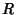have a Chord at distance. The Area enclosed by the Chord, shown as the shaded region in the above figure, is then(1)

But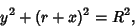(2)

so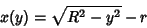(3)

and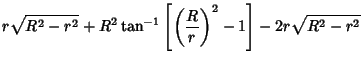(4)

Checking the limits, when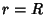,and when,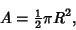(5)